# Understanding Database Normalization with Example

Normalization or database normalization is a process to organize the data into database tables. To make a good database design, you have to follow Normalization practices. Without normalization, a database system might be slow, inefficient and might not produce the expected result. Normalization reduces data redundancy and inconsistent data dependency.

To make a good database design, you must know the normal forms and their best uses. In this article, we will discuss the normal forms.

## Normal Forms: Types of Normalization

We organize the data into database tables by using normal forms of rules or conditions. Normal forms help us to make a good database design. Generally, we organize the data up to the third normal form. We rarely use the fourth and fifth normal form.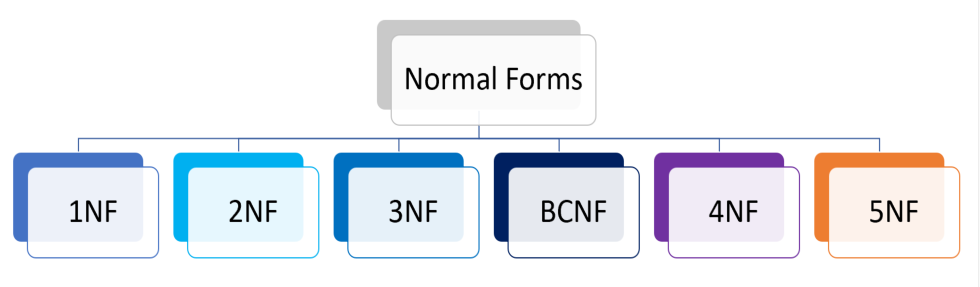To understand normal forms consider the following unnormalized database table. Now we will normalize the data of below table using normal forms.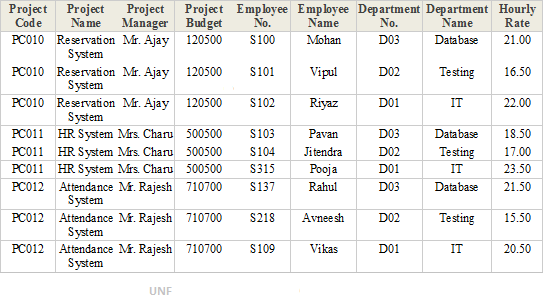1. ## First Normal Form (1NF)

A database table is said to be in 1NF if it contains no repeating fields/columns. The process of converting the UNF table into 1NF is as follows:

1. Separate the repeating fields into new database tables along with the key from the unnormalized database table.

2. The primary key of new database tables may be a composite key

1NF of above UNF table is as follows: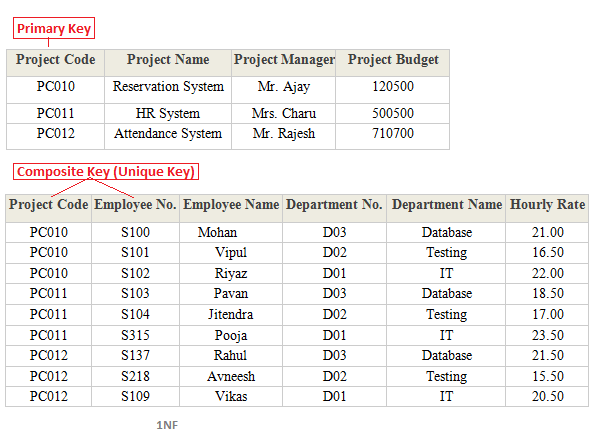2. ## Second Normal Form (2NF)

A database table is said to be in 2NF if it is in 1NF and contains only those fields/columns that are functionally dependent(means the value of the field is determined by the value of another field(s)) on the primary key. In 2NF we remove the partial dependencies of any non-key field.

The process of converting the database table into 2NF is as follows:
1. Remove the partial dependencies(A type of functional dependency where a field is only functionally dependent on the part of primary key) of any non-key field.

2. If field B depends on field A and vice versa. Also for a given value of B, we have only one possible value of A and vice versa, Then we put the field B into new database table where B will be the primary key and also marked as a foreign key in a parent table.

2NF of above 1NF tables is as follows: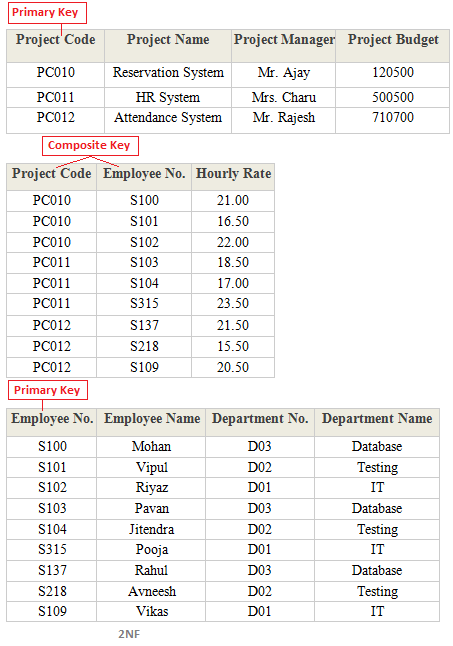3. ## Third Normal Form (3NF)

A database table is said to be in 3NF if it is in 2NF and all non-keys fields should be dependent on primary key or We can also say a table to be in 3NF if it is in 2NF and no fields of the table are transitively functionally dependent on the primary key. The process of converting the table into 3NF is as follows:

1. Remove the transitive dependencies(A type of functional dependency where a field is functionally dependent on the Field that is not the primary key. Hence its value is determined, indirectly by the primary key )

2. Make a separate table for transitive dependent Field.

3NF of above 2NF tables is as follows: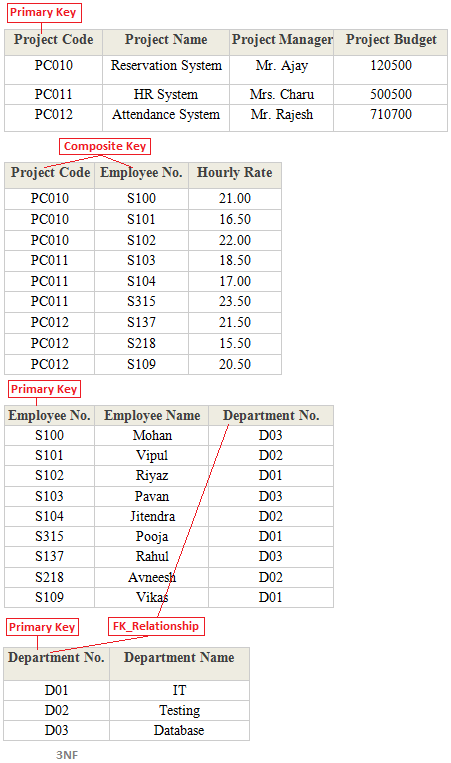4. ## Boyce Code Normal Form (BCNF)

A database table is said to be in BCNF if it is in 3NF and contains each and every determinant as a candidate key. The process of converting the table into BCNF is as follows:

1. Remove the nontrivial functional dependency.

2. Make a separate table for the determinants.

BCNF of below table is as follows: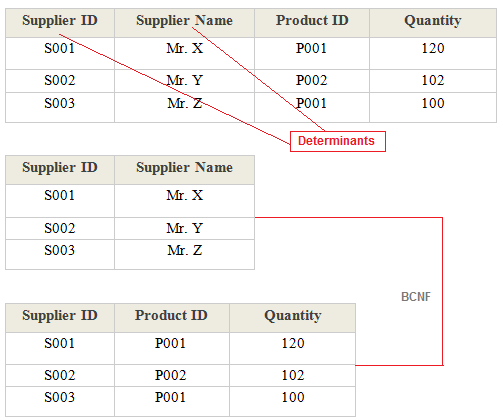5. ## Fourth Normal Form (4NF)

A database table is said to be in 4NF if it is in BCNF and primary key has a one-to-one relationship to all non-keys fields or We can also say a table to be in 4NF if it is in BCNF and contains no multi-valued dependencies. The process of converting the table into 4NF is as follows:

1. Remove the multivalued dependency.

2. Make a separate table for multivalued Fields.

4NF of below table is as follows: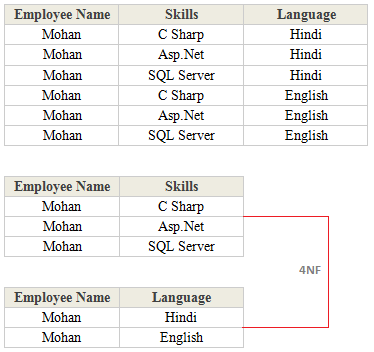6. ## Fifth Normal Form (5NF)

A database table is said to be in 5NF if it is in 4NF and contains no redundant values or We can also say a table to be in 5NF if it is in 4NF and contains no join dependencies. The process of converting the table into 5NF is as follows:

1. Remove the join dependency.

2. Break the database table into smaller and smaller tables to remove all data redundancy.

5NF of below table is as follows: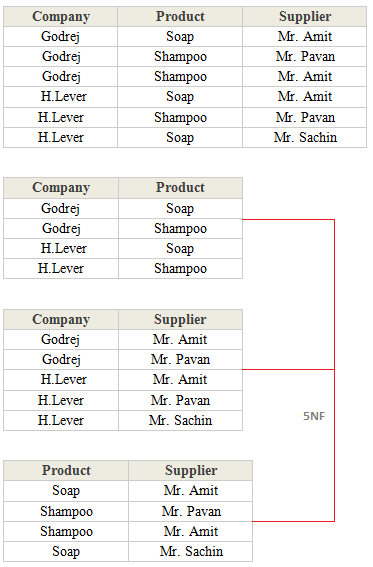Read More Articles Related to SQL Server
##### Summary